# Topic 12: Quantum and nuclear physics (HL)

See the guide for this topic.

## 12.1 – The interaction of matter with radiation

• ### Photons

• Einstein proposed that light consists of particles called photons.
• Quantum refers to the smallest discrete amount of something. A photon is a quantum of electromagnetic radiation (light).
• Photons exhibit wave properties under refraction or interference.
• Photons exhibit wave properties under its emission or absorption.• A photon’s energy (E) is proportional to its frequency (f) and is given by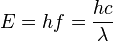where h is Planck’s constant, c is the speed of light, and λ is its wavelength (electromagnetic wave).

• ### The photoelectric effect

• Photoelectric effect refers to the emission of electrons from a metal surface as a result of the absorption of electromagnetic wave energy.Factor Effect Intensity Affects the number of electrons ejected. Frequency There exists a minimum frequency (depending on the material) below which no electrons are ejected. Affects the maximum KE of ejected electrons
• An example of the photoelectric effect on a sample metal surface.Incident electromagnetic waves with lower frequency have a smaller chance of inducing the photoelectric effect.

• Why does the intensity of light affect the number of ejected electrons?

The number of photons per unit time in the incident light is proportional to the light intensity.

An increase in the intensity of the incident light allows a higher number of photon-electron interactions. Therefore, more electrons are ejected.

• Why is there a minimum frequency below which no electrons are ejected?

There exists a minimum energy below which electrons would not be ejected from the metal. This minimum energy level depends on the metal in use and is called the work function (φ).

Since E=hf, φ=hf0 where f0 is called the threshold frequency.

• How does the frequency of the incident light affect the maximum kinetic energy of the ejected electrons?

The work function corresponds to the potential energy which binds the electron to the nucleus.

Since total energy = potential energy + kinetic energy,which may be represented on graph by the following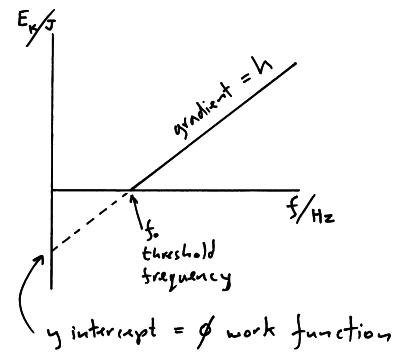• ### Matter waves

• The De Broglie hypothesis suggests that all matter exhibits wave-like properties. In particular, the momentum of a particle is related to its wavelength where the De Broglie wavelength may be deduced by the following formula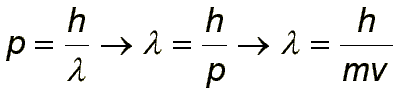where p is momentum, h is Planck’s constant, λ is wavelength, m is mass, and v is velocity.

• The term “wave-particle duality” refers to matter acting as both waves and particles.

• ### Pair production and pair annihilation

All matters have their antimatter counterparts which resemble their corresponding matter in every way except for the sign of their charge and the direction of their spin.

Pair production

When a high energy photon collides with a nucleus, it makes a pair of electron and positron (electron antimatter) and gives kinetic energy to each particle.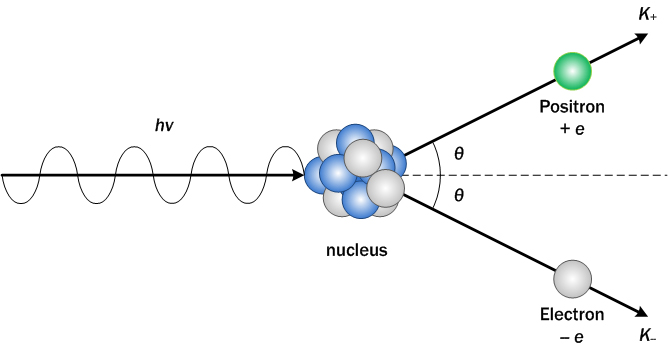Pair annihilation

When matter collides with its corresponding antimatter, they annihilate one another with the conservation of energy, momentum, and charge.The positron (+e) collides with the electron (-e), annihilating each other into two photons with exactly opposite directions and the same amount of momentum.

• ### Quantization of angular momentum in the Bohr model for hydrogen

• Bohr developed a model for hydrogen that was able to explain the emission and absorption spectra of hydrogen.
• His model assumed discrete orbital paths in which electrons orbit the nucleus through, the same way planets orbit stars.
• The orbits were quantized in terms of their allowable angular momentum (rotational momentum).
• Therefore, the orbital radii and energies are also quantized.
• The energy of the orbit is the energy required to ionize (remove) an electron and can be given through the following equation in relation to the order of orbit (n)• When the electrons are excited, they jump to higher energy orbits and eventually drop back down to a more stable orbit by releasing excess energy by the form of light. The energy of the light released is therefore equal to the difference in energy of the two orbits.

• ### The wave function

By quantum physics, all particles do not have a defined position until they are observed. Instead, all particles are described as “a wave function”.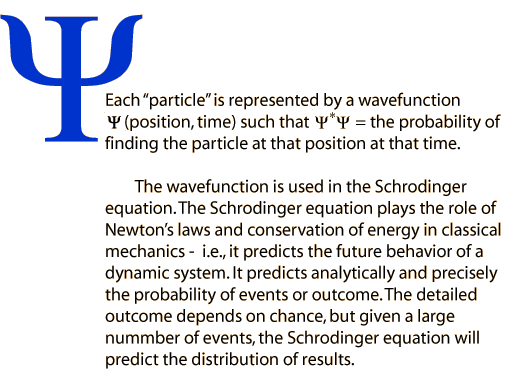TL;DR: The wave function gives the probability of finding a particle at a given point which is given by the square of the amplitude of the wave function at that location.

• ### The uncertainty principle for energy and time and position and momentum

The Heisenberg uncertainty principle states that

• If the energy state only lasts for a brief period of time, its energy is uncertain.• Position and momentum cannot be measured simultaneously with precision. The more precisely the position is determined, the less precisely the momentum is known, and vice versa.• ### Tunnelling, potential barrier and factors affecting tunnelling probability

• Imagine throwing a ball at a wall and having it disappear the instant before making contact and appearing on the other side. The wall remains intact and the ball did not break through it. Believe it or not, there is a finite (if extremely small) probability that this even would occur. This phenomenon is called quantum tunnelling.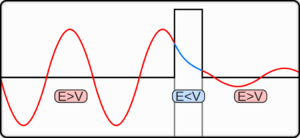• The position of a particle is described as a wave function (see previous section).
• From the graph above, the observable particle is most likely to be at the position where its wave function has the largest amplitude. However, although the amplitude of the wave function will decay exponentially, since the wave function does not reach an amplitude of zero, the wave function can exit the barrier. Once the wave function exits the barrier, its amplitude no longer decays. This means that a particle has a certain probability of bouncing off a barrier and a certain probability of passing through the other side.
 Factor Effect towards tunnelling probability Increase barrier length Decrease Increase particle mass Decrease
• This explains how tunnelling is frequent in nanoscale but negligible at the macroscopic level.

## 12.2 – Nuclear physics

• ### Rutherford scattering and nuclear radius

Rutherford’s undergraduate students, Geiger and Marsden, bombarded a sheet of gold foil by alpha particles.The alpha particles passed through the gold foil in most cases, a small percentage of alpha particles were deflected by small angles of deflection, and an even smaller percentage of alpha particles were deflected by large angles of deflection.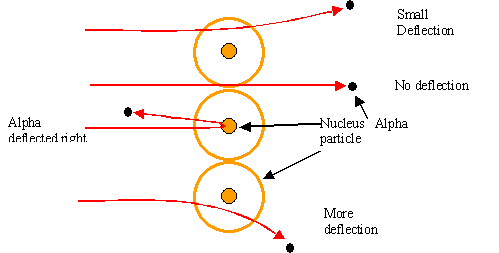Rutherford thus deduced that the atom consists of a small compact positive nucleus (where alpha particles deflect by large angles) with a majority of volume existing as empty space (where alpha particles pass right through).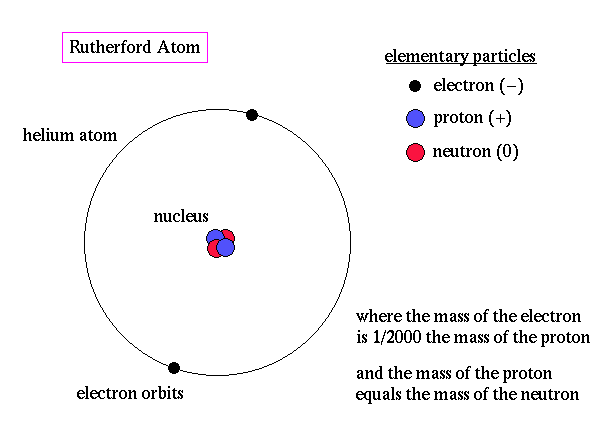• ### Nuclear energy levels

• In the same way electrons can move between discrete energy levels, the nucleus of an atom can too.
• Atoms that decay through gamma decay emit distinct frequencies of gamma rays which correspond to distinct energy levels.• ### The neutrino

• A neutrino is a type of lepton. Since they have no electrical charge or strong charge, most neutrinos do not react with other particles and pass right through earth with no interaction.
• Neutrinos are produced in many particle decays, such as in beta decay. When a neutron at rest (zero momentum) decays by releasing a proton and an electron, because of the law of conservation of momentum, the resultant products of decay must have a total momentum of zero, which the observed proton and electron clearly does not portray. Therefore, we suggest the presence of another particle to balance the momentum – by the release of an antineutrino (neutrino antimatter). This was confirmed by experimentation.• Neutrinos were produced in great abundance in the early universe and rarely interact with matter. This may suggest that neutrinos contribute to the total mass of the universe and affects its expansion.

• ### The law of radioactive decay and the decay constant

Apart from half-lives (see topic 7), the activity of radioactive decay can also be shown exponentially by the law of radioactive decay.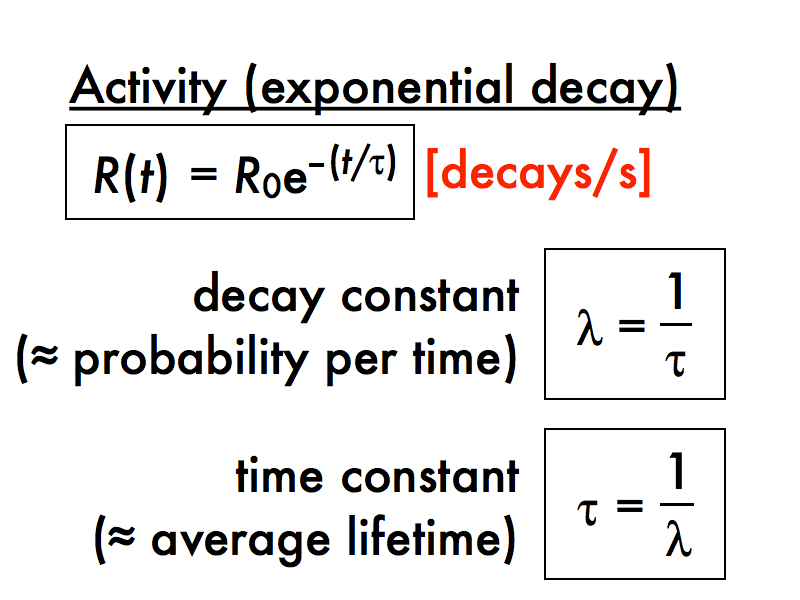• The decay constant (λ) represents the probability of decay of a nucleus per unit time and is dependent on the type of element.